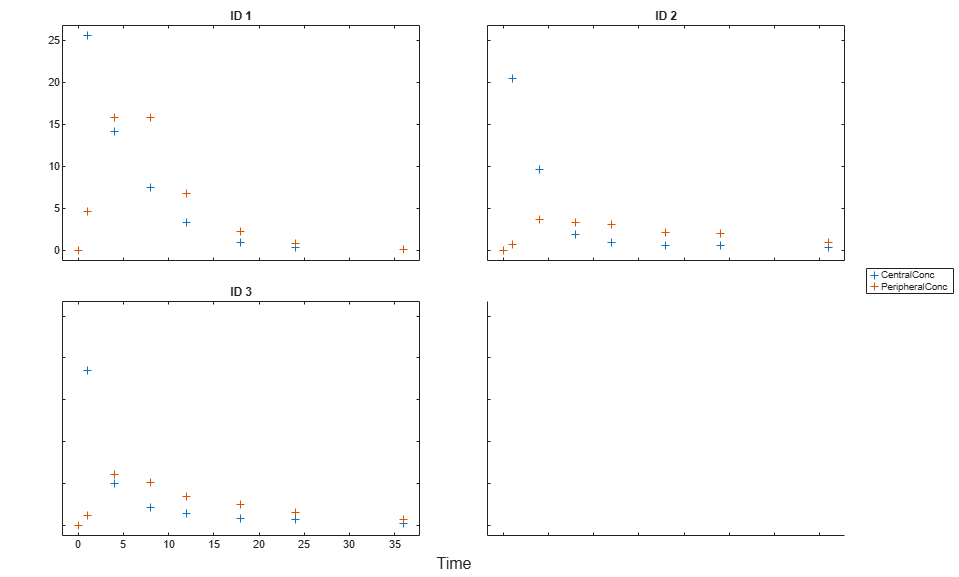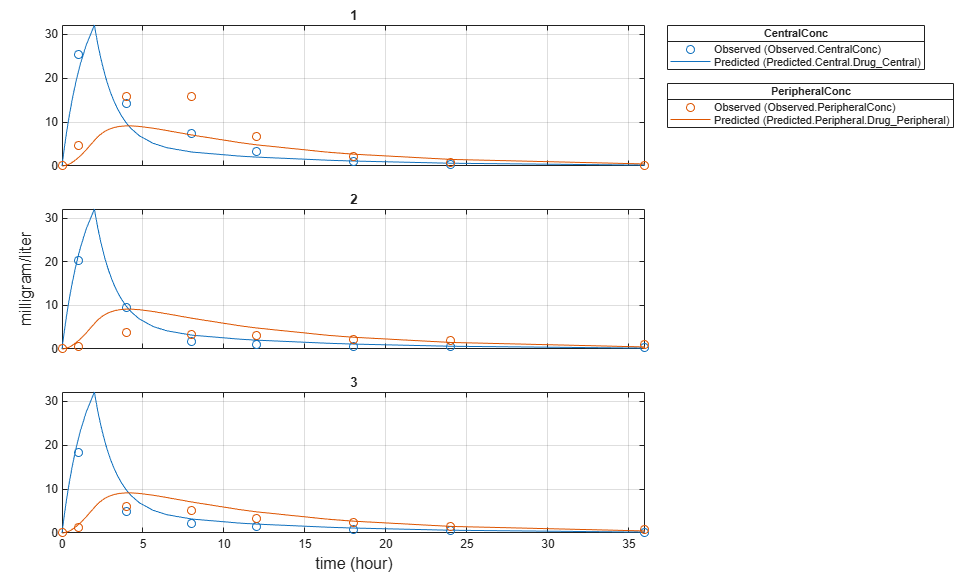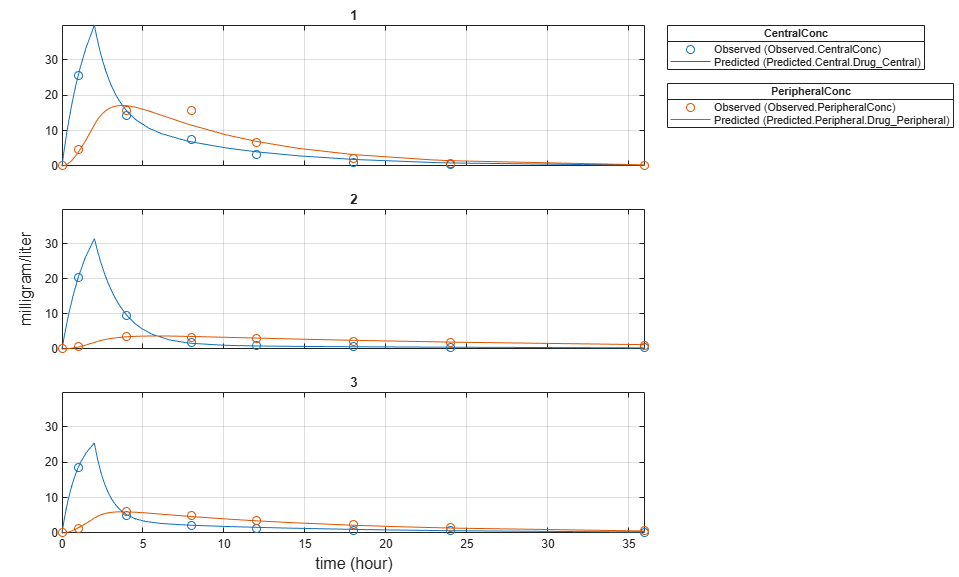## Fit a Two-Compartment Model to PK Profiles of Multiple Individuals

This example shows how to estimate pharmacokinetic parameters of multiple individuals using a two-compartment model.

Suppose you have drug plasma concentration data from three individuals that you want to use to estimate corresponding pharmacokinetic parameters, namely the volume of central and peripheral compartment (`Central`, `Peripheral`), the clearance rate (`Cl_Central`), and intercompartmental clearance (`Q12`). Assume the drug concentration versus the time profile follows the biexponential decline ${C}_{t}=A{e}^{-at}+B{e}^{-bt}$, where Ct is the drug concentration at time t, and a and b are slopes for corresponding exponential declines.

The synthetic data set contains drug plasma concentration data measured in both central and peripheral compartments. The data was generated using a two-compartment model with an infusion dose and first-order elimination. These parameters were used for each individual.

`Central``Peripheral``Q12``Cl_Central`
Individual 11.900.680.240.57
Individual 22.106.050.360.95
Individual 31.704.210.460.95

The data is stored as a table with variables `ID`, `Time`, `CentralConc`, and `PeripheralConc`. It represents the time course of plasma concentrations measured at eight different time points for both central and peripheral compartments after an infusion dose.

```load('data10_32R.mat') ```

Convert the data set to a `groupedData` object which is the required data format for the fitting function `sbiofit` for later use. A `groupedData` object also lets you set independent variable and group variable names (if they exist). Set the units of the `ID`, `Time`, `CentralConc`, and `PeripheralConc` variables. The units are optional and only required for the `UnitConversion` feature, which automatically converts matching physical quantities to one consistent unit system.

```gData = groupedData(data); gData.Properties.VariableUnits = {'','hour','milligram/liter','milligram/liter'}; gData.Properties ```
```ans = struct with fields: Description: '' UserData: [] DimensionNames: {'Row' 'Variables'} VariableNames: {'ID' 'Time' 'CentralConc' 'PeripheralConc'} VariableDescriptions: {} VariableUnits: {1x4 cell} VariableContinuity: [] RowNames: {} CustomProperties: [1x1 matlab.tabular.CustomProperties] GroupVariableName: 'ID' IndependentVariableName: 'Time' ```

Create a trellis plot that shows the PK profiles of three individuals.

```sbiotrellis(gData,'ID','Time',{'CentralConc','PeripheralConc'},... 'Marker','+','LineStyle','none'); ```Use the built-in PK library to construct a two-compartment model with infusion dosing and first-order elimination where the elimination rate depends on the clearance and volume of the central compartment. Use the configset object to turn on unit conversion.

```pkmd = PKModelDesign; pkc1 = addCompartment(pkmd,'Central'); pkc1.DosingType = 'Infusion'; pkc1.EliminationType = 'linear-clearance'; pkc1.HasResponseVariable = true; pkc2 = addCompartment(pkmd,'Peripheral'); model = construct(pkmd); configset = getconfigset(model); configset.CompileOptions.UnitConversion = true; ```

Assume every individual receives an infusion dose at time = 0, with a total infusion amount of 100 mg at a rate of 50 mg/hour. For details on setting up different dosing strategies, see Doses in SimBiology Models.

```dose = sbiodose('dose','TargetName','Drug_Central'); dose.StartTime = 0; dose.Amount = 100; dose.Rate = 50; dose.AmountUnits = 'milligram'; dose.TimeUnits = 'hour'; dose.RateUnits = 'milligram/hour'; ```

The data contains measured plasma concentrations in the central and peripheral compartments. Map these variables to the appropriate model species, which are `Drug_Central` and `Drug_Peripheral`.

```responseMap = {'Drug_Central = CentralConc','Drug_Peripheral = PeripheralConc'}; ```

The parameters to estimate in this model are the volumes of central and peripheral compartments (`Central` and `Peripheral`), intercompartmental clearance `Q12`, and clearance rate `Cl_Central`. In this case, specify log-transform for `Central` and `Peripheral` since they are constrained to be positive. The `estimatedInfo` object lets you specify parameter transforms, initial values, and parameter bounds (optional).

```paramsToEstimate = {'log(Central)','log(Peripheral)','Q12','Cl_Central'}; estimatedParam = estimatedInfo(paramsToEstimate,'InitialValue',[1 1 1 1]); ```

Fit the model to all of the data pooled together, that is, estimate one set of parameters for all individuals. The default estimation method that `sbiofit` uses will change depending on which toolboxes are available. To see which estimation function `sbiofit` used for the fitting, check the `EstimationFunction` property of the corresponding results object.

```pooledFit = sbiofit(model,gData,responseMap,estimatedParam,dose,'Pooled',true) ```
```pooledFit = OptimResults with properties: ExitFlag: 3 Output: [1x1 struct] GroupName: [] Beta: [4x3 table] ParameterEstimates: [4x3 table] J: [24x4x2 double] COVB: [4x4 double] CovarianceMatrix: [4x4 double] R: [24x2 double] MSE: 6.6220 SSE: 291.3688 Weights: [] LogLikelihood: -111.3904 AIC: 230.7808 BIC: 238.2656 DFE: 44 DependentFiles: {1x3 cell} EstimatedParameterNames: {'Central' 'Peripheral' 'Q12' 'Cl_Central'} ErrorModelInfo: [1x3 table] EstimationFunction: 'lsqnonlin' ```

Plot the fitted results versus the original data. Although three separate plots were generated, the data was fitted using the same set of parameters (that is, all three individuals had the same fitted line).

```plot(pooledFit); ```Estimate one set of parameters for each individual and see if there is any improvement in the parameter estimates. In this example, since there are three individuals, three sets of parameters are estimated.

```unpooledFit = sbiofit(model,gData,responseMap,estimatedParam,dose,'Pooled',false); ```

Plot the fitted results versus the original data. Each individual was fitted differently (that is, each fitted line is unique to each individual) and each line appeared to fit well to individual data.

```plot(unpooledFit); ```Display the fitted results of the first individual. The MSE was lower than that of the pooled fit. This is also true for the other two individuals.

```unpooledFit(1) ```
```ans = OptimResults with properties: ExitFlag: 3 Output: [1x1 struct] GroupName: 1 Beta: [4x3 table] ParameterEstimates: [4x3 table] J: [8x4x2 double] COVB: [4x4 double] CovarianceMatrix: [4x4 double] R: [8x2 double] MSE: 2.1380 SSE: 25.6559 Weights: [] LogLikelihood: -26.4805 AIC: 60.9610 BIC: 64.0514 DFE: 12 DependentFiles: {1x3 cell} EstimatedParameterNames: {'Central' 'Peripheral' 'Q12' 'Cl_Central'} ErrorModelInfo: [1x3 table] EstimationFunction: 'lsqnonlin' ```

Generate a plot of the residuals over time to compare the pooled and unpooled fit results. The figure indicates unpooled fit residuals are smaller than those of pooled fit as expected. In addition to comparing residuals, other rigorous criteria can be used to compare the fitted results.

```t = [gData.Time;gData.Time]; res_pooled = vertcat(pooledFit.R); res_pooled = res_pooled(:); res_unpooled = vertcat(unpooledFit.R); res_unpooled = res_unpooled(:); plot(t,res_pooled,'o','MarkerFaceColor','w','markerEdgeColor','b') hold on plot(t,res_unpooled,'o','MarkerFaceColor','b','markerEdgeColor','b') refl = refline(0,0); % A reference line representing a zero residual title('Residuals versus Time'); xlabel('Time'); ylabel('Residuals'); legend({'Pooled','Unpooled'}); ```This example showed how to perform pooled and unpooled estimations using `sbiofit`. As illustrated, the unpooled fit accounts for variations due to the specific subjects in the study, and, in this case, the model fits better to the data. However, the pooled fit returns population-wide parameters. If you want to estimate population-wide parameters while considering individual variations, use `sbiofitmixed`.